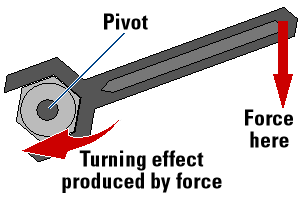Home PHYSICS TOPIC 4: FORCES IN EQUILIBRIUM | PHYSICS FORM 1

# TOPIC 4: FORCES IN EQUILIBRIUM | PHYSICS FORM 1

148
1
SHARE### TOPIC 4: FORCES IN EQUILIBRIUM | PHYSICS FORM 1

Moment of a Force

The Effects of Turning Forces
Explain the effects of turning forces
force is a push or pull which when applied to a body it will change its state either by stopping it if it was in motion or making it move if it was at rest.
If a body under the action of a net externalforceis allowed to rotate about a pivot, the body will tend to turn in the direction of the applied force.Examples of turning effect of force:
• A person pushing a swing will make the swing rotate about its pivot.
• A worker applies force to a spanner to rotate a nut.
• A person removes a bottle’s cork by pushing down the bottle opener’s lever.
• Force is applied to a door knob and the door swings open about its hinge.
• A driver can turn a steering wheel by applying force on its rim.
The Moment of Force
Determine the moment of force
a point is the turning effect of the force about that point. The change
of state of a body can appear in several forms and the most common form
is the turning effect which is referred to as moment of a force.
The unit for a force isNewton, Nwhile that for moment of a force isNewton-meter, Nm.
Moment = Force, F x Perpendicular distance, x.
Moment = Fx
Consider the diagram below;Moment of W₁ about the fulcrum. = W₁d₁
Moment of W₂ about fulcrum = W₂d₂
The Principle of Moments
State the principle of moments
The Principle of moments states that:”If
a body is in equilibrium under a force which lie in one plane, the sum
of the clockwise moments is equal to the sun of the anticlockwise
moments about any point in that plane.”
Activity 1
Experiment.
Aim: To determine the moment of a force.
Materials and apparatus:Meter rule, several different weights, inelastic cotton thread, knife edge and a marker pen.
Procedures
• Balance the meter rule horizontally on a knife edge.
• Mark a balance point as C. Use the marker pen to do that.
• Suspend
a meter rule from a fixed axis through C. Suspend unequal weights W₁
and W₂ from the meter rule by using thin cotton threads.
• Adjust the distance d₁ and d₂ of the weights W₁ and W₂ from C until the meter rule balance.
• Repeat the experiment five times using different values of W₁ and W₂.Record the results on the table as shown below.
 W₁(g) W₂(g) d₁(cm) d₂(cm) W₁ d₁ (gcm) W₂ d₂ (gcm)
Observation:In each case it will be found that W₁ d₁ is equal to W₂ d₂.
The Principle of Moment in Daily Life
Apply the principle of moment in daily life
Moment of a force is used in the following:
• Is applied by a hand to unscrew a stopper on the bottle.
• Is applied by a spanner to unscrew a nut on a bottle.
• Turning a steering wheel of a car.

#### 1 COMMENT

1. Very good article post.Much thanks again. Really Great.